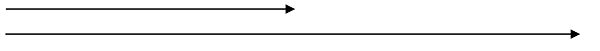# Scalar Multiplication of Vector | Linear Algebra using Python

Linear Algebra using Python: Here, we are going to learn how find the scalar multiplication of vectors?
Submitted by Anuj Singh, on May 10, 2020

Prerequisite: Linear Algebra | Defining a Vector

Linear algebra is the branch of mathematics concerning linear equations by using vector spaces and through matrices. In other words, a vector is a matrix in n-dimensional space with only one column. In linear algebra, there are two types of multiplication:

1. Scalar Multiplication
2. Cross Multiplication

In a scalar product, each component of the vector is multiplied by the same a scalar value. As a result, the vector’s length is increased by scalar value.

For example: Let a vector a = [4, 9, 7], this is a 3 dimensional vector (x,y and z)

So, a scalar product will be given as b = c*a

Where c is a constant scalar value (from the set of all real numbers R). The length vector b is c times the length of vector a.## Python code for Scalar Multiplication of Vector

```# Vectors in Linear Algebra Sequnce (5)
# Scalar Multiplication of Vector

def scalar(c, a):
b = []
for i in range(len(a)):
b.append(c*a[i])
return b

a = [3, 5, -5, 8] # This is a 4 dimensional vector

print("Vector a = ", a)
c = int(input("Enter the value of scalar multiplier: "))

# The vector b will have the same dimensions
# but the overall magnitute is c times a
print("Vector (b = c*a) = ", scalar(c, a))
```

Output

```Vector a =  [3, 5, -5, 8]
Enter the value of scalar multiplier: 3
Vector (b = c*a) =  [9, 15, -15, 24]
```

Preparation

What's New

Top Interview Coding Problems/Challenges!